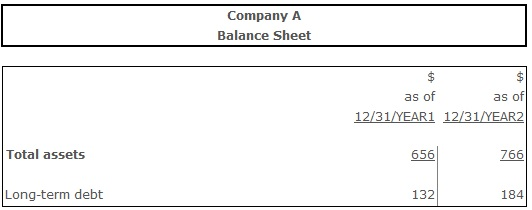# Long-Term Debt Ratio

Long-Term Debt Ratio – a ratio, measuring the percentage of company’s total assets financed with long-term debt.

## Formula(s):

Long-Term Debt Ratio = Long-Term Debt ÷ Total Assets

## Example:Long-Term Debt Ratio (Year 1) = 132 ÷ 656= 0,20

Long-Term Debt Ratio (Year 2) = 184 ÷ 766 = 0,24

## Conclusion:

Calculations show that long-term debt ratio was relatively stable over the period of year 1-year 2. Year 2 witnessed a very slight 20% to 24% increase of the long-term debt share in company’s source of finance.